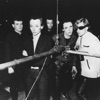# Wowwy

## Magazine

this is what i'm on a about ,
its all about (wowwy's x5)
this is what i'm on a about ,
its all about (wowwy's x5)
this is what i'm on a about ,
its all about (wowwy's x5)
this is what i'm on a about ,
its all about (wowwy's x5)
this is what i'm on a about ,
its all about (wowwy's x5)
this is what i'm on a about ,
its all about (wowwy's x5)
this is what i'm on a about ,
its all about (wowwy's x5)
this is what i'm on a about ,
its all about (wowwy's x5)
this is what i'm on a about ,
its all about (wowwy's x5)
this is what i'm on a about ,
its all about (wowwy's x5)
this is what i'm on a about ,
its all about (wowwy's x5)
this is what i'm on a about ,
its all about (wowwy's x5)
this is what i'm on a about ,
its all about (wowwy's x5)
this is what i'm on a about ,
its all about (wowwy's x5)
this is what i'm on a about ,
its all about (wowwy's x5)
this is what i'm on a about ,
its all about (wowwy's x5)
this is what i'm on a about ,
its all about (wowwy's x5)
this is what i'm on a about ,
its all about (wowwy's x5)
this is what i'm on a about ,
its all about (wowwy's x5)
this is what i'm on a about ,
its all about (wowwy's x5)
this is what i'm on a about ,
its all about (wowwy's x5)
this is what i'm on a about ,
its all about (wowwy's x5)
this is what i'm on a about ,
its all about (wowwy's x5)
this is what i'm on a about ,
its all about (wowwy's x5)
this is what i'm on a about ,
its all about (wowwy's x5)
this is what i'm on a about ,
its all about (wowwy's x5)
this is what i'm on a about ,
its all about (wowwy's x5)
this is what i'm on a about ,
its all about (wowwy's x5)
this is what i'm on a about ,
its all about (wowwy's x5)
this is what i'm on a about ,
its all about (wowwy's x5)
this is what i'm on a about ,
its all about (wowwy's x5)
this is what i'm on a about ,
its all about (wowwy's x5)
this is what i'm on a about ,
its all about (wowwy's x5)
this is what i'm on a about ,
its all about (wowwy's x5)
this is what i'm on a about ,
its all about (wowwy's x5)
this is what i'm on a about ,
its all about (wowwy's x5)
this is what i'm on a about ,
its all about (wowwy's x5)
this is what i'm on a about ,
its all about (wowwy's x5)
this is what i'm on a about ,
its all about (wowwy's x5)
this is what i'm on a about ,
its all about (wowwy's x5)
this is what i'm on a about ,
its all about (wowwy's x5)
this is what i'm on a about ,
its all about (wowwy's x5)
this is what i'm on a about ,
its all about (wowwy's x5)
this is what i'm on a about ,
its all about (wowwy's x5)
this is what i'm on a about ,
its all about (wowwy's x5)
this is what i'm on a about ,
its all about (wowwy's x5)
this is what i'm on a about ,
its all about (wowwy's x5)
this is what i'm on a about ,
its all about (wowwy's x5)
this is what i'm on a about ,
its all about (wowwy's x5)
this is what i'm on a about ,
its all about (wowwy's x5)
this is what i'm on a about ,
its all about (wowwy's x5)
this is what i'm on a about ,
its all about (wowwy's x5)
this is what i'm on a about ,
its all about (wowwy's x5)
this is what i'm on a about ,
its all about (wowwy's x5)
this is what i'm on a about ,
its all about (wowwy's x5)
this is what i'm on a about ,
its all about (wowwy's x5)
this is what i'm on a about ,
its all about (wowwy's x5)
this is what i'm on a about ,
its all about (wowwy's x5)
this is what i'm on a about ,
its all about (wowwy's x5)
this is what i'm on a about ,
its all about (wowwy's x5)
this is what i'm on a about ,
its all about (wowwy's x5)
this is what i'm on a about ,
its all about (wowwy's x5)
this is what i'm on a about ,
its all about (wowwy's x5)
this is what i'm on a about ,
its all about (wowwy's x5)
this is what i'm on a about ,
its all about (wowwy's x5)
this is what i'm on a about ,
its all about (wowwy's x5)
this is what i'm on a about ,
its all about (wowwy's x5)
this is what i'm on a about ,
its all about (wowwy's x5)
this is what i'm on a about ,
its all about (wowwy's x5)
this is what i'm on a about ,
its all about (wowwy's x5)
this is what i'm on a about ,
its all about (wowwy's x5)